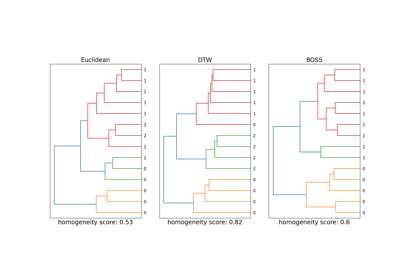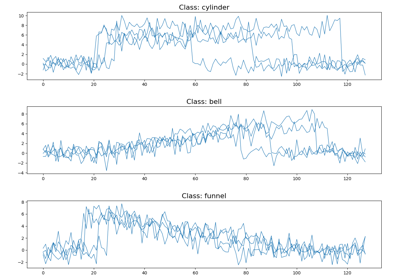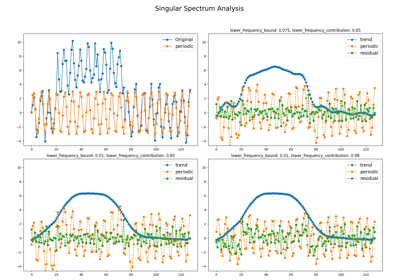# pyts.datasets.make_cylinder_bell_funnel¶

pyts.datasets.make_cylinder_bell_funnel(n_samples=30, weights=None, shuffle=True, random_state=None, return_params=False)[source]

Make a Cylinder-Bell-Funnel dataset.

The classes are coded with the following meaning:

• 0: Cylinder
• 1: Bell
• 2: Funnel
Parameters: n_samples : int (default = 30) The number of time series. weights : list of floats or None (default=None) The proportions of samples assigned to each class. If None, then classes are balanced. Note that if len(weights) == 2, then the last class weight is automatically inferred. More than n_samples samples may be returned if the sum of weights exceeds 1. shuffle : bool (default = True) If True, shuffle the samples. return_params : bool (default = False) If True, a dictionary containing the parameters used to make the time series is returned. random_state : int, RandomState instance or None (default = None) Determines random number generation for dataset creation. Pass an int for reproducible output across multiple function calls. X : array, shape = (n_samples, 128) Generated time series. y : array, shape = (n_samples,) Generated class labels. Labels have the following meaning: 0: Cylinder 1: Bell 2: Funnel params : dict The parameters used to generate the data. The keys are ‘a’, ‘b’, ‘eta’ and ‘epsilon’ and the values are the corresponding values. Only returned if return_params=True.

Notes

The time series are generated from the following distributions:where,is an integer-valued uniform random variable on the inverval,is an integer-valued uniform distribution on the inveral,andare standard normal variables,is the characteristic function on the interval.,, andstand for “cylinder”, “bell”, and “funnel” respectively.

References

  N. Saito, “Local feature extraction and its application using a library of bases”. Ph.D. thesis, Department of Mathematics, Yale University, 1994.

Examples

>>> import numpy as np
>>> from pyts.datasets import make_cylinder_bell_funnel
>>> X, y = make_cylinder_bell_funnel()
>>> X.shape
(30, 128)
>>> print(np.bincount(y))
[10 10 10]


## Examples using pyts.datasets.make_cylinder_bell_funnel¶Time Series Clustering with DTW and BOSS

Time Series Clustering with DTW and BOSSMaking a Cylinder-Bell-Funnel dataset

Making a Cylinder-Bell-Funnel datasetTrend-Seasonal decomposition with Singular Spectrum Analysis

Trend-Seasonal decomposition with Singular Spectrum Analysis